# JavaScript原生对象属性和方法详解——Math对象

September 18, 2013
author: feeldesignstudio.com

Math对象主要是用来执行一些数学任务，介绍它们的同时，也帮大家回忆一下数学知识。

## E

Math.E 属性代表算术常量 e，即自然对数的底数，其值近似于 2.71828。

## LN2

LN2 属性就是 loge2，即 2 的自然对数，其值近似于 0.69314718055994528623。

## LN10

LN10 属性就是 loge10，即 10 的自然对数，其值近似于 2.3025850929940459011。

## LOG2E

LOG2E 属性就是 log2e，即以 2 为底 e 的对数，其值近似于 1.442695040888963387。

## PI

PI 属性就是 π，也就是常说的圆周率，即圆的周长和它的直径之比。这个值近似为 3.141592653589793。

## SQRT2

SQRT2 属性返回 2 的平方根。这个值近似为 1.4142135623730951。

## SQRT1_2

SQRT1_2 属性返回 2 的平方根的倒数。这个值近似为 0.7071067811865476。

``````console.log(Math.E);        //2.718281828459045
console.log(Math.LN2);      //0.6931471805599453
console.log(Math.LN10);     //2.302585092994046
console.log(Math.LOG2E);    //1.4426950408889634
console.log(Math.LOG10E);   //0.4342944819032518
console.log(Math.PI);       //3.141592653589793
console.log(Math.SQRT1_2);  //0.7071067811865476
console.log(Math.SQRT2);    //1.4142135623730951
``````

## abs(x)

abs() 方法可返回数的绝对值。

abs() 是静态方法，调用的方式是Math.abs(x)，Math对象的方法一般都是静态方法，调用方式均为 Math.方法名(参数)，就不一一说明了。

``````Math.abs('-1');     // 1
Math.abs(-2);       // 2
Math.abs(null);     // 0
Math.abs("string"); // NaN
Math.abs();         // NaN
``````

## ceil(x)

ceil() 方法可对一个数进行上舍入，方法执行的是向上取整计算。

``````Math.ceil(.95);   // 1
Math.ceil(4);     // 4
Math.ceil(7.004); // 8
``````

## floor(x)

floor() 方法可对一个数进行下舍入，方法执行的是向下取整计算。

``````Math.floor(.95);   // 0
Math.floor(4);     // 4
Math.floor(7.004); // 7
``````

## round(x)

round() 方法可把一个数字舍入为最接近的整数

``````Math.round(20.49);  //20
Math.round(20.5);   //21
Math.round(-20.5);  //-20
Math.round(-20.51); //-21
``````

``````function roundNumber(number, digits) {
if (arguments.length == 1)
return Math.round(number);

var multiple = Math.pow(10, digits);
var rndedNum = Math.round(number * multiple) / multiple;
return rndedNum;
}
``````

## exp(x)

exp() 方法可返回 e 的 x 次幂的值。

``````Math.exp(1);  //2.718281828459045
Math.exp(2);  //7.3890560989306495
``````

## log(x)

log() 方法可返回一个数的自然对数。

``````Math.log(10);   //2.302585092994046
Math.log(0);    //-Infinity
Math.log(-1);   //NaN
Math.log("a");  //NaN
``````

## max(x…)

max() 方法可返回两个或多个指定的数中带有较大的值的那个数。

``````Math.max(7.25,7.30,7.301);  //7.301
Math.max();                 //-Infinity
Math.max(1,"a");            //NaN
``````

## min(x…)

min() 方法可返回指定的数字中带有最低值的数字。

``````Math.min(7.25,7.30,7.301);  //7.25
Math.min();                 //Infinity
Math.min(1,"a");            //NaN
``````

## pow(x,y)

pow() 方法可返回 x 的 y 次幂的值。

``````Math.pow(2,2);    //4
Math.pow(2,2,3);  //4
Math.pow(2,"a");  //NaN
Math.pow(2);      //NaN
Math.pow();       //NaN
Math.pow(1000000,1000000); //Infinity
``````

## sqrt(x)

sqrt() 方法可返回一个数的平方根。

``````Math.pow(2,2);  //4
Math.sqrt(4);   //2
``````

## random()

random() 方法可返回介于 0 ~ 1 之间（不包括1）的一个随机数。

``````//获取min和max之间的随机数
function getRandomArbitrary(min, max) {
return Math.random() * (max - min) + min;
}

//获取min和max之间的随机整数
function getRandomInt(min, max) {
return Math.floor(Math.random() * (max - min + 1) + min);
}
``````

``````Math.randomRange = function(min, max) {
if (min && max) {
return (min + Math.floor(Math.random() * (max - min + 1)));
} else if (min) {
return (Math.floor(Math.random() * min + 1))
} else {
return (Math.floor(Math.random() * 101));
}
}
``````

``````generateGUID =
(typeof(window.crypto) != 'undefined' && typeof(window.crypto.getRandomValues) != 'undefined')
? function () {
var buf = new Uint16Array(8);
window.crypto.getRandomValues(buf);
var S4 = function (num) {
var ret = num.toString(16);
while (ret.length < 4) {
ret = "0" + ret;
}
return ret;
};
return (S4(buf) + S4(buf) + "-" + S4(buf) + "-" + S4(buf) + "-" + S4(buf) + "-" + S4(buf) + S4(buf) + S4(buf));
}
: function () {
return 'xxxxxxxx-xxxx-4xxx-yxxx-xxxxxxxxxxxx'.replace(/[xy]/g, function (c) {
var r = Math.random() * 16 | 0, v = c == 'x' ? r : (r & 0x3 | 0x8);
return v.toString(16);
});
};
``````

## 三角函数和反三角函数

### 1、直角三角形中的三角函数：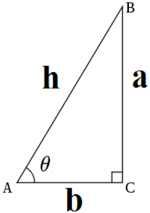• θ的正弦是对边与斜边的比值：sinθ = a / h
• θ的余弦是邻边与斜边的比值：cosθ = b / h
• θ的正切是对边与邻边的比值：tanθ = a / b
• θ的余切是邻边与对边的比值：cotθ = b / a
• θ的正割是斜边与邻边的比值：secθ = h / b
• θ的余割是斜边与对边的比值：cscθ = h / a

### 2、任意角的三角函数计算方式：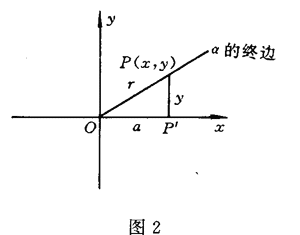sinα = y / r cosα = x / r tanα = y / x

• 第一象限内任何一个角的三角函数值都是“+”；
• 第二象限内只有正弦、余割是“+”，其余全部是“-”；
• 第三象限内只有正切、余切函数是“+”，其余函数是“-”；
• 第四象限内只有余弦、正割是“+”，其余全部是“-”。

### 3、弧度制与角度制的转换：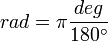### 4、常用的三角函数公式：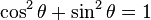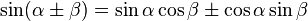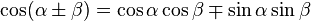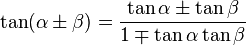### 5、反三角函数：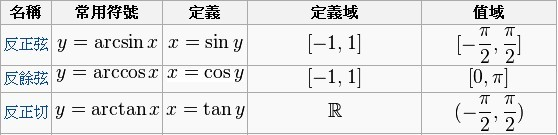## cos(x)

cos() 方法可返回一个数字的余弦值。

## sin(x)

sin() 方法可返回一个数字的正弦。

## tan(x)

tan() 方法可返回一个表示某个角的正切的数字。

## acos(x)

acos() 方法可返回一个数的反余弦。

## asin(x)

asin() 方法可返回一个数的反正弦值。

## atan(x)

atan() 方法可返回数字的反正切值。

## atan2(y,x)

atan2() 方法可返回从 x 轴到点 (x,y) 之间的角度。

Tips: 评论也可以匿名哦，不一定注册的，只需要勾选匿名评论(I'd rather post as a guest)即可